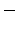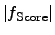# 4.1 Basic Issues on Optimization

From a mathematical point of view, optimization is a rather straight forward task for standard problems, for instance if a set of linear or non-linear equations is considered. However, the optimization of TCAD problems has to deal with highly complex score functions (objective functions). These score functions include pre-processing steps like geometry construction, meshing, and process simulation, the actual simulation with its manifolds of different models, and at the end, there is a set of post-processing steps to obtain the appropriate quantities from the simulation results. From these data sets, the quality has to be determined with respect to a user-defined reference. These sets of equations and models cannot be sufficiently determined to obtain a best strategy for an optimization because the different input parameters can cause to switch the behavior of the models due to different operation conditions. Therefore, the global behavior of the simulation results changes. Hence, the convergence of the simulation cannot be guaranteed for all parameter sets of the domain of the input parameters. For instance, changing the geometry might require a different meshing algorithm to obtain a sufficiently good resolution for reasonable simulation results. On top of that, numerical difficulties may arise within every part of the optimization loop.

From a mathematical point of view, the exchange of the score functionwith its negative valuestransforms a minimization to a maximization of, showing the same convergence criteria . However, some implicit assumption of certain properties of the score function yields a limited range of applications. For instance, a very common procedure is to use the absolute value of the score function. As a consequence, the information about the sign of the score function vanishes. Whether this is the case or not should be denoted in the particular optimizer's documentation. However, the user has to be aware of these different procedures within the optimizer and to take it into account by using appropriate user-defined score functions.

Improvements of the optimization algorithm in terms of convergence can be achieved by iterative methods  (cf. NEWTON's4.5iteration scheme as a propaedeutic example). However, these methods are limited by a certain domain in which the optimization algorithm shows the property of high convergence. With a starting point outside of this defined domain, the convergence speed can often not be determined, and sometimes the convergence of the optimization algorithm is not even guaranteed. An additional challenge in optimization is that there is no knowledge whether the objective function is twice continuously differentiable because many optimization algorithm assume this property.

There are several different optimization methods, where each having its benefits and drawbacks. The optimization methods can be dived into several groups: local and global An additional classification of optimization strategies separates the techniques in incomplete, asymptotically complete, complete, and rigorously complete optimization algorithms. An optimal optimizer would combine the benefits of the iterative optimization algorithms and the genetic and randomized search, certain techniques for stochastic, heuristic, and genetic approaches  can be combined to build new optimization strategies in order to increase the probability of finding a good optimum within a short period of time. Other improvements to speed up the optimization is to use parallel optimization  and to select the appropriate optimization algorithm .

Typical optimizations take a lot of time. Therefore, reducing execution time for optimization is very important to provide results within reasonable time. There are several principal methods to speed up a optimization run:

• Parallelization of the optimization algorithm:
This methods is advantageous when a huge amount of data has to be processed in each optimization iteration step. For instance a complex response surface method (RSM) optimization requires a huge amount of computational power and memory to calculate the parameters and coefficients for the next iteration. In this case, a parallelization makes sense because the computational effort and execution time is of the same order of magnitude as the evaluation of a particular parameter. However, the parallelization of the optimization algorithm often does not decrease the overall optimization time all that much, because the evaluation of particular parameter sets usually takes much longer (minutes to weeks) than the calculation of the next parameter sets (seconds to minutes). Hence, the execution time of the optimizer program can often be neglected compared to the time which is necessary to evaluate the parameters by its score function, which includes the entire simulation sequence.

• Parallelization of the evaluations of parameter sets:
The evaluations of parameter sets can be generally computed concurrently because the proposed parameter sets from the optimizer do not depend on each other. For instance, if a optimizer has to calculate a gradient in a certain point of the parameter domain, a certain amount of necessary parameter sets for evaluation are submitted concurrently. Hence, these parameter sets can also be evaluated in parallel and, if possible, on different computational nodes. However, if the calculation of gradients are computational expensive, gradient-free optimization algorithm are advantageous.

• Parallelization of the solvers in the simulation tools:
To parallelize the solver in the simulation tools increases the performance of each parameter evaluation during the optimization. However, the influence of whether the simulation tools use parallel solvers is very limited because many vendors distribute their software with fixed capabilities and features.

• Selection of a good optimization strategy and an appropriate score function:
To choose the appropriate optimization strategy is one of the most critical tasks during the optimization setup because the strategy defines several additional parameters and at the end also the convergence speed. However, the score function has to be chosen according to the type of the problem and according to the selected optimization strategy to provide fast and accurate optimization results. If additional constraint functions are necessary, they have to be aligned to the score function to avoid numerical problems within the optimizer. If, for instance, the numerical values are too different which can cause problems with the precision which yields numerical noise and therefore wrong optimization results.

The main goal of an optimization is to find the parameter set that yields the global optimum in a minimum number of iterations. However, this search is often very complex and would require to check the complete parameter space in all dimensions which often leads to serious problems in terms of finite resources of computational power and time. To overcome this type of problem, the optimization can be split into three major parts where the first part deals with the separation of the most significant and less significant parameters. This part requires a good knowledge of the problem class which has to be optimized. Once the parameters are separated with respect to their importance and significance, the optimization of the significant parameters can be started. This leads to certain good parameter sets which can be fine tuned in the third part where all parameters are included in the optimization. Appropriate constraint functions and close intervals for the parameters can drastically reduce the computational effort for the parameter search.

Subsections
Stefan Holzer 2007-11-19